# Difference between revisions of "Separately continuous not implies continuous"

## Statement

### For a function of two variables at a point

It is possible to have the following: a function$f$ of two variables$x,y$ that is separately continuous in both variables$x,y$ at the point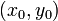$(x_0,y_0)$, but is not a continuous function at$(x_0,y_0)$.

### For a function of two variables overall

It is possible to have the following: a function$f$ of two variables$x,y$ that is separately continuous in both variables$x,y$ everywhere on$\R^2$, but is not continuous everywhere on$\R^2$ (i.e., there exist points where it is not continuous.

## Proof

### Example

We give a single example that illustrates both versions of the statement.

Consider the function: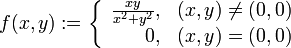$f(x,y) := \left\lbrace \begin{array}{rl} \frac{xy}{x^2 + y^2}, & (x,y) \ne (0,0) \\ 0, & (x,y) = (0,0) \\\end{array}\right.$

It's clear that$f$ is continuous, as well as separately continuous, at all points other than$(0,0)$. At the point$(0,0)$, we calculate the limits along the$x$-direction and$y$-direction:

• The limit along the$x$-direction is$\lim_{x \to 0} \frac{(x)(0)}{x^2 + 0^2} = \lim_{x \to 0} 0 = 0$. This coincides with the value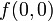$f(0,0)$.
• The limit along the$y$-direction is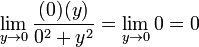$\lim_{y \to 0} \frac{(0)(y)}{0^2 + y^2} = \lim_{y \to 0} 0 = 0$. This coincides with the value$f(0,0)$.

Thus, the function$f$ is separately continuous in both$x$ and$y$ at the point$(0,0)$. Since we already established that it's separately continuous everywhere else, we obtain that it is separately continuous on all of$\R^2$.

On the other hand,$f$ is not continuous at$(0,0)$. To see this, consider the limit along the line$y = x$. Setting$y = x$, we get that the limit is:$\lim_{x \to 0} \frac{xx}{x^2 + x^2} = \lim_{x \to 0} \frac{x^2}{2x^2} = \lim_{x \to 0}\frac{1}{2} = \frac{1}{2} \ne f(0,0)$

Note that if the function were indeed continuous at$(0,0)$, the limit along every direction would equal the value at the point, so this shows that the function is not continuous at$(0,0)$.

### Idea behind example

The secret behind the example can be better understood using polar coordinates, though this is not necessary to understand the example itself. In polar coordinates, we can rewrite$f(x,y) = g(r,\theta)$ where:$g(r,\theta) = \left \lbrace \begin{array}{rl} \frac{1}{2}\sin(2\theta), & r \ne 0\\ 0, & r = 0 \\\end{array}\right.$

For$\theta$ a multiple of$\pi/2$ (which covers both the$x$- and the$y$-directions, both positive and negative),$g(r,\theta) = 0$ identically. Hence, the limit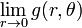$\lim_{r \to 0} g(r,\theta)$ is zero. For other values of$\theta$, on the other hand,$g(r,\theta) = \frac{1}{2}\sin(2\theta)$ identically along the line and the limit$\lim_{r \to 0} g(r,\theta)$ is also the number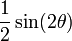$\frac{1}{2}\sin(2\theta)$, which is nonzero. Thus, the function is not continuous along any slanting direction, and hence is not a continuous function at the origin (which in polar coordinates we'd call the pole).